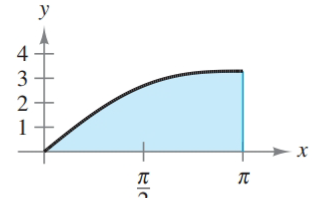Chapter 8.5, Problem 49E### Calculus: An Applied Approach (Min...

10th Edition
Ron Larson
ISBN: 9781305860919

#### Solutions

Chapter
Section### Calculus: An Applied Approach (Min...

10th Edition
Ron Larson
ISBN: 9781305860919
Textbook Problem
1 views

# Finding Area by Integration In Exercises 47-52, find the area of the region. See Example 7. y = x + sin xTo determine

To calculate: The area bounded by the graph of y=x+sinx.

Explanation

Given Information:

The provided graph of y=x+sinx can be shown as,

Formula used:

The formula of definite integral of absinudu.

absinudu=[cosu]ab

The formula of definite integral of abundu.

abundu=[un+1n+1]ab

Calculation:

From provided diagram, the interval [a,b] will be [0, π]. Therefore, area bounded by graph of y=x+sinx can be calculated as,

0π(x+sinx)dx=0πxdx+0πsinxdx

Integrate with the help of above integration formulae

### Still sussing out bartleby?

Check out a sample textbook solution.

See a sample solution

#### The Solution to Your Study Problems

Bartleby provides explanations to thousands of textbook problems written by our experts, many with advanced degrees!

Get Started

#### Find more solutions based on key concepts